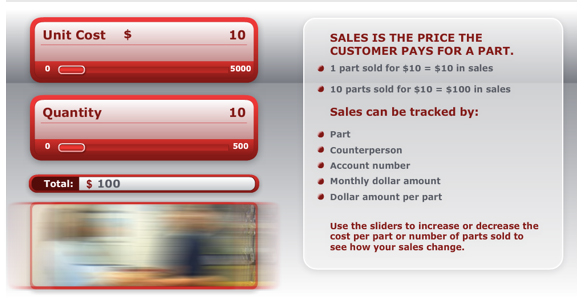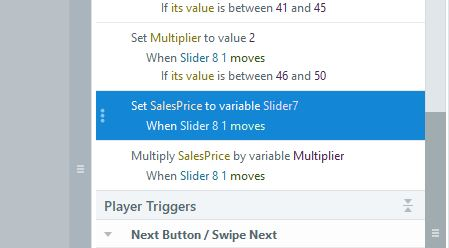# Sliders and Multiplication

Wondering if someone can point me in the right direction..

I have to create something like this:The user changes the unit cost and quantity and the total changes as per those 2 units.

Trying to figure out how to would set up different variables and link them with the slider and total box. Any pointers would be great.

###### 15 RepliesHi Shawna,

The attached example will show you how this can be achieved.  Set up two sliders, which generate their own variables and then set up a third variable to enable the calculation to be carried out.  The reason the triggers are replicated for each slider is so that the calculation can be adjusted whichever slider the user moves first and irrespective of whether the other slider is moved or not.

If you have any queries, just get back to me here.

Hope this helps.Hi Shawna,

Hopefully I have understood your question and the attached may be what you are looking for.

Firstly I noticed that Slider 6 1 appeared to reference variable Slider3, but I assumed this was meant to be Slider6 and have adjusted accordingly.

With regards to the example, I have added two new variables (ProfitLock and Profit), which are controlled by the two new buttons at the bottom. The sequence of events now is that you move the top slider and the bottom slider to show your respective prices and obtain a total at the bottom. Once you are happy with the profit, click on the Lock In Profit button and you will see that locked in profit registers between the two buttons. Now, if you adjust the Sales Price slider, the Cost slider will also adjust in order to maintain the same profit. If you now wish to change your profit, simply click on the Unlock Profit button and start all over again.

The way this works is simply by using the two buttons to adjust a True/False variable (ProfitLock), which is then used as a condition for setting the value of the second slider.

Hope this is what you are after.  If you have any further problems, just get back to me here.Thank you! That helps!

I do have one more question - I have created another slider diagram

This one has 4 sections

"Cost of sales" which values are from 100-500

"Gross profit" which has a set of 8 values
0-15% = \$1.17
16-20% = \$1.25
21-25% = \$1.33
26-30% = \$1.42
31-35% = \$1.54
36-40% = \$1.67
41-45% = \$1.81
46-50% = \$2.00

"Multiplier" which shows the dollar amount of what percentage is selected in "Gross profit" above

and

"Sales price" which is supposed to show the "Cost of sale" multiplied by the "Multiplier" - but I cant quite figure out how to get this number.

Both cost of sale and multiplier has variables, but no matter what I try, I cant get that to populate.Hi Shawna,

This is an easy fix as you have simply left out one trigger. At the point at which you multiply SalesPrice by Multiplier, your SalesPrice variable is set to its initial value of zero and hence the answer will always be zero. What you have to do first is to set SalesPrice equal to the Cost of Sales (Slider7) and then carry out the multiplication:Wondering if you could help me with one other thing.

When the Cost of Sale slider is moved, the Multiplier and Sales Price do not change, only when the Gross Profit slider is moved, do they populate. I have tried adjusting the variable when the first slider moves, but it doesn't seem to work for me.

Would you be able to have a look at the file and see what I am doing wrong?

Thanks a bunch.Hi Shawna,

A couple of minor issues, but a simple fix (see attached update).

First of all, I noticed that you had doubled up on two of your triggers under Slider 8 1 and so have removed the bottom two (just a tidy-up as they don't actually affect the outcome):Secondly, the problem you were experiencing was due to the fact that your initial trigger under Slider 7 1 was set to variable Slider8 instead of Slider7 (highlighted in blue below).  I have also added three additional triggers at the start (highlighted in yellow below), which set up an initial Multiplier and give you a set of meaningful figures in your calculator (instead of zeros) when the slide first appears (note, you need to set up the Multiplier before carrying out the calculation):If you have any other queries, just get back to me here.